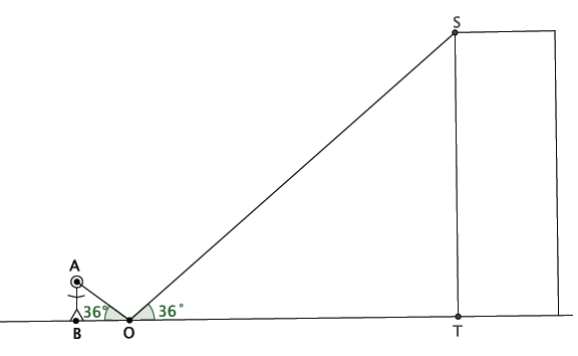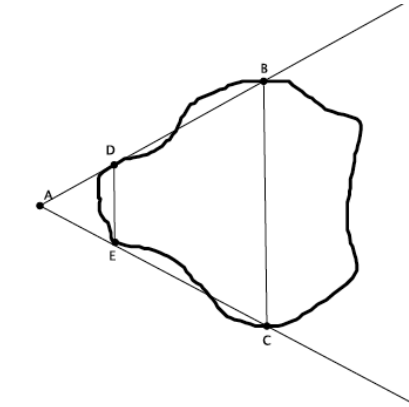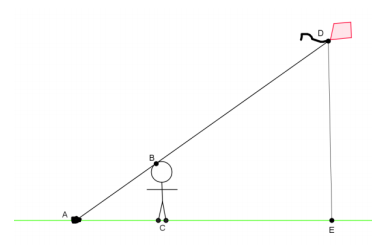Match Fishtank is now Fishtank Learning!

# Transformations and Angle Relationships

## Objective

Use properties of similar triangles to model and solve real-world problems.

## Common Core Standards

### Core Standards

?

• 8.G.A.4 — Understand that a two-dimensional figure is similar to another if the second can be obtained from the first by a sequence of rotations, reflections, translations, and dilations; given two similar two-dimensional figures, describe a sequence that exhibits the similarity between them.

?

• 7.RP.A.2

• 7.RP.A.3

## Criteria for Success

?

1. Model real-world situations using similar triangles (MP.4).
2. Use ratio reasoning to find missing measurements in real-world applications of similar triangles.

## Tips for Teachers

?

• Students have not yet covered the angle-angle criterion for similar triangles, but they do not need that for this lesson. Students can understand the similarity between the triangles using dilations and transformations. For example, in Anchor Problem #1, students can see how the small triangle can be reflected over the vertical line through Point $O$ and then dilated from Point $O$ to map to the larger triangle.
• Calculators may be used in this lesson to keep the focus on the conceptual understanding.

#### Remote Learning Guidance

If you need to adapt or shorten this lesson for remote learning, we suggest prioritizing Anchor Problem 1 or 2 (benefit from worked examples). Find more guidance on adapting our math curriculum for remote learning here.

#### Fishtank Plus

• Problem Set
• Student Handout Editor
• Vocabulary Package

## Anchor Problems

?

### Problem 1

You want to determine the approximate height of one of the buildings in the city. You are told that if you place a mirror some distance from yourself so that you can see the top of the building in the mirror, then you can indirectly measure the height using similar triangles. Let point $O$ be the location of the mirror so that the person shown can see the top of the building.If you know that the distance from eye-level straight down to the ground is 5.3 feet, the distance from the person to the mirror is 7.2 feet, and the distance from the mirror to the base of the building is 1,742.8 feet, then what is the height of the building?

#### References

EngageNY Mathematics Grade 8 Mathematics > Module 3 > Topic B > Lesson 12Teacher Version: Exercise 1

Grade 8 Mathematics > Module 3 > Topic B > Lesson 12 of the New York State Common Core Mathematics Curriculum from EngageNY and Great Minds. © 2015 Great Minds. Licensed by EngageNY of the New York State Education Department under the CC BY-NC-SA 3.0 US license. Accessed Dec. 2, 2016, 5:15 p.m..

Modified by Fishtank Learning, Inc.

### Problem 2

A geologist wants to determine the distance across the widest part of a nearby lake. The geologist marked off specific points around the lake so that the line containing ${\overline{{DE}}}$ would be parallel to the line containing ${\overline{{BC}}}$. The segment ${BC}$ is selected specifcally because it is the widest part of the lake. The segment ${DE}$ is selected specifically because it is a short enough distance to easily measure.The geologist has made the following measurements: $\left | {DE} \right |$=5 feet, ${\left | AE \right |}$= 7 feet, and ${\left | EC \right |}$ = 15 feet. Can she determine the length across the widest part of the lake?

#### References

EngageNY Mathematics Grade 8 Mathematics > Module 3 > Topic B > Lesson 12Teacher Version: Exercise 2

Grade 8 Mathematics > Module 3 > Topic B > Lesson 12 of the New York State Common Core Mathematics Curriculum from EngageNY and Great Minds. © 2015 Great Minds. Licensed by EngageNY of the New York State Education Department under the CC BY-NC-SA 3.0 US license. Accessed Dec. 2, 2016, 5:15 p.m..

Modified by Fishtank Learning, Inc.

## Problem Set

?The following resources include problems and activities aligned to the objective of the lesson that can be used to create your own problem set.

• Spiral in review problems from earlier in the unit.
• Challenge: Write your own real-world problem, ensuring it has all the information needed to solve. Swap with a peer and solve your peer's problem.

?

Henry thinks he can figure out how high his kite is while flying it in the park. First, he lets out 150 feet of string and ties the string to a rock on the ground. Then he moves from the rock until the string touches the top of his head. He stands up straight, forming a right angle with the ground. He wants to find out the distance from the ground to his kite. He draws the following diagram to illustrate what he has done.1. Does Henry have enough information to so far to use similar triangles to find the height of his kite? Explain.
2. Henry knows that he is ${5 \frac{1}{2}}$ feet tall. Henry measures the string from the rock to his head and finds it to be $8$ feet. Determine what the height of the kite is.

#### References

EngageNY Mathematics Grade 8 Mathematics > Module 3 > Topic B > Lesson 12Exit Ticket

Grade 8 Mathematics > Module 3 > Topic B > Lesson 12 of the New York State Common Core Mathematics Curriculum from EngageNY and Great Minds. © 2015 Great Minds. Licensed by EngageNY of the New York State Education Department under the CC BY-NC-SA 3.0 US license. Accessed Dec. 2, 2016, 5:15 p.m..

Modified by Fishtank Learning, Inc.

?